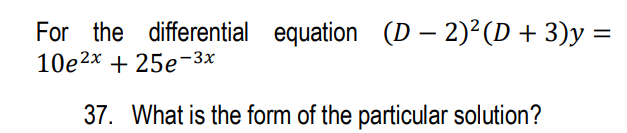### Create an Account

Home / Questions / For the differential equation (D – 2)2(D + 3)y = 10e2x + 25e-3x 37. What is the form of th...

# For the differential equation (D – 2)2(D + 3)y = 10e2x + 25e-3x 37. What is the form of the particular solution?

For the differential equation (D – 2)2(D + 3)y = 10e2x + 25e-3x 37. What is the form of the particular solution?May 12 2021 View more View LessSubscribe To Get Solution